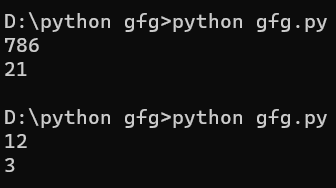# TCS Coding Practice Question | Sum of Digits of a number

Given a number, the task is to find the Sum of Digits of this number using Command Line Arguments

Examples:

```Input: num = 687
Output: 21

Input: num = 12
Output: 3```

Approach:

• Since the number is entered as Command line Argument, there is no need for a dedicated input line
• Extract the input number from the command line argument
• This extracted number will be in string type.
• Convert this number into integer type and store it in a variable, say num
• Declare a variable to store the sum and set it to 0
• Repeat the next two steps till the number is not 0
• Get the rightmost digit of the number with help of remainder ‘%’ operator by dividing it with 10 and add it to sum.
• Divide the number by 10 with help of ‘/’ operator
• Print or return the sum

Program:

## C

 `// C program to find` `// the sum of digits of a number` `// using command line arguments`   `#include ` `#include /* atoi */`   `// Function to Find the sum of digits` `int` `findSumOfDigits(``int` `num)` `{`   `    ``// Variable to store the` `    ``// the sum of digits` `    ``int` `sum = 0;`   `    ``// Traverse through the number digit by digit` `    ``while` `(num > 0) {`   `        ``// Add the last digit of num` `        ``// to the sum` `        ``sum = sum + (num % 10);`   `        ``// Remove the last digit from the num` `        ``num = num / 10;` `    ``}`   `    ``// Return the sum` `    ``return` `sum;` `}`   `// Driver code` `int` `main(``int` `argc, ``char``* argv[])` `{`   `    ``int` `num;`   `    ``// Check if the length of args array is 1` `    ``if` `(argc == 1)` `        ``printf``(``"No command line arguments found.\n"``);` `    ``else` `{`   `        ``// Get the command line argument and` `        ``// Convert it from string type to integer type` `        ``// using function "atoi( argument)"` `        ``num = ``atoi``(argv);`   `        ``// Find the sum of digits and print it` `        ``printf``(``"%d\n"``, findSumOfDigits(num));` `    ``}` `    ``return` `0;` `}`

## Java

 `// Java program to find` `// the sum of digits of a number` `// using command line arguments`   `class` `GFG {`   `    ``// Function to Find the sum of digits` `    ``public` `static` `int` `findSumOfDigits(``int` `num)` `    ``{`   `        ``// Variable to store the` `        ``// the sum of digits` `        ``int` `sum = ``0``;`   `        ``// Traverse through the number digit by digit` `        ``while` `(num > ``0``) {`   `            ``// Add the last digit of num` `            ``// to the sum` `            ``sum = sum + (num % ``10``);`   `            ``// Remove the last digit from the num` `            ``num = num / ``10``;` `        ``}`   `        ``// Return the sum` `        ``return` `sum;` `    ``}`   `    ``// Driver code` `    ``public` `static` `void` `main(String[] args)` `    ``{`   `        ``// Check if length of args array is` `        ``// greater than 0` `        ``if` `(args.length > ``0``) {`   `            ``// Get the command line argument and` `            ``// Convert it from string type to integer type` `            ``int` `n = Integer.parseInt(args[``0``]);`   `            ``// Find the sum of digits and print it` `            ``System.out.println(findSumOfDigits(n));` `        ``}` `        ``else` `            ``System.out.println(``"No command line "` `                               ``+ ``"arguments found."``);` `    ``}` `}`

## Python

 `# Python program to find the sum of digits of a number`   `def` `findSumOfDigits(num):` `      ``sum` `=` `0` `      ``# Traverse through the number digit by digit` `      ``while` `(num > ``0``):` `            ``# Add the last digit of num to the sum` `            ``sum` `=` `sum` `+` `(num ``%` `10``)` `            ``# Remove the last digit from the num` `            ``num ``=` `num ``/``/` `10` `      ``return` `sum`   `# take the input ` `num``=``(``input``())` `# convert into integer type` `n``=``int``(num)` `# check the length of number` `if` `len``(num)>``0``:` `        ``print``(findSumOfDigits(n))` `else``:` `       ``print``(``"No command line"``)` `            `  `         `

## C++

 `#include ` `#include // for atoi function` `using` `namespace` `std;`   `// Function to find the sum of digits` `int` `findSumOfDigits(``int` `num)` `{` `    ``int` `sum = 0;` `    ``while` `(num > 0) {` `        ``sum += num % 10; ``// add the last digit to the sum` `        ``num /= 10; ``// remove the last digit from num` `    ``}` `    ``return` `sum;` `}`   `// Main driver code` `int` `main(``int` `argc, ``char``* argv[])` `{` `    ``int` `num;`   `    ``// Check if at least one command line argument was provided` `    ``if` `(argc == 1) {` `        ``cout << ``"No command line arguments found."` `<< endl;` `        ``return` `0; ``// exit program` `    ``}`   `    ``// Convert the first command line argument to an integer` `    ``num = ``atoi``(argv);`   `    ``// Calculate the sum of digits of the number and output the result` `    ``cout << findSumOfDigits(num) << endl;`   `    ``return` `0; ``// exit program` `}`

Output:Time complexity: O(logn)

Auxiliary Space: O(1)

Whether you're preparing for your first job interview or aiming to upskill in this ever-evolving tech landscape, GeeksforGeeks Courses are your key to success. We provide top-quality content at affordable prices, all geared towards accelerating your growth in a time-bound manner. Join the millions we've already empowered, and we're here to do the same for you. Don't miss out - check it out now!

Previous
Next